﻿ Equation of State for the Study of Temperature Dependence of Volume Thermal Expansion of Nanomaterials

### Equation of State for the Study of Temperature Dependence of Volume Thermal Expansion of Nanomateria...OPEN ACCESSPEER-REVIEWED

## Equation of State for the Study of Temperature Dependence of Volume Thermal Expansion of Nanomaterials

Mahipal Singh1,, Madan Singh2

1Department of Physics, R.H. Govt. P. G. College, Kashipur, Uttarakhand, India

2Department of Physics and Electronics, National University of Lesotho, Roma 180, Lesotho

### Abstract

A new integral form of equation of state (IFEOS) for volume thermal expansion has been established and used to study the volume thermal expansion of nanomaterials under the effect of temperature. A wide variety of nanomaterials such as fullerene (C60), 20 nm-Ni, 15 nm-(80Ni+20Fe), n-ZnO, n-TiO2 and n-NiO has been considered to analyze the effect of temperature on them. The results obtained have been compared with available experimental data as well as other theoretical approaches. Excellent agreement between theory and available experimental data supports the validity of newly established integral form of equation of state (IFEOS) for nanomaterials.

### At a glance: Figures

12
Prev Next

• Singh, Mahipal, and Madan Singh. "Equation of State for the Study of Temperature Dependence of Volume Thermal Expansion of Nanomaterials." American Journal of Nanomaterials 2.2 (2014): 26-30.
• Singh, M. , & Singh, M. (2014). Equation of State for the Study of Temperature Dependence of Volume Thermal Expansion of Nanomaterials. American Journal of Nanomaterials, 2(2), 26-30.
• Singh, Mahipal, and Madan Singh. "Equation of State for the Study of Temperature Dependence of Volume Thermal Expansion of Nanomaterials." American Journal of Nanomaterials 2, no. 2 (2014): 26-30.

 Import into BibTeX Import into EndNote Import into RefMan Import into RefWorks

### 1. Introduction

Over the past decade, the nanomaterials have been the subject of enormous interest. These materials, remarkable for their extremely small size, have the potential for wide ranging industrial, biomedical, electronic and engineering applications. Therefore, it is not just a matter of great interest but also a necessity to study the thermodynamic properties of nanocrystalline materials. Investigation of the behavior of nanomaterials under high temperature can provide valuable information about their intrinsic micro structural characteristics. Nanomaterials differ significantly from their bulk counterparts primarily because of their small size. The study of nanocrystalline materials with particle dimensions less than 100 nm is an active area of research in Physics, Chemistry and Engineering . Nanomaterials are usually very sensitive to external parameters such as temperature etc.

In the 1985, hollow carbon nanospheres were discovered. These spheres were called buckyballs or fullerenes, in the honor of architect and futurist Buckminster Fuller, who designed a geodesic dome with geometry similar to that found on the molecular level in fullerenes . The basic C60 structure consists of 60 carbon atoms that link together to form a hollow cage-like structure. The structure consists of 32 faces of which 20 are hexagons and 12 are pentagons. Of these, no two pentagons share a common side. Several physical properties of bulk fullerenes have been studied by Fischer et al.  and Lundin et al.  but less literature is available for a single fullerene C60.

Nanocrystalline nickel (n-Ni), an important nanocrystalline metal, has been the subject of considerable experimental and theoretical work in past years [5, 6]. Nickel is a transition ferromagnetic 3d metal that is widely used as catalyst and thus initiated work by several authors to better understand its behavior at extreme conditions [7, 8]. Zinc oxide (ZnO) is an inorganic compound and occurs as a white powder which belongs to the family of wide-band-gap (3.3 eV) semiconductors with strong ionic character of chemical bonds and a well known material with many potential applications eg. Piezoelectric transducer, UV laser, transparent conducting films and efficient sunblock coatings for human skin protection [9, 10, 11, 12]. TiO2 is also a wide band gap (3.2 eV) semiconducting material. It is a promising nonlinear optical material due to its transparency and large refractive index. Several natural polymorphs of TiO2 exist, the most common are anatase, rutile and brookite. Rutile is the stable phase, whereas anatase and brookite are meta stable. These are numerous major technological applications of this material in various areas such as in the pigments, plastics, cosmetics, electronics, in oil and air purification. It is useful in nitrogen fixation as well as dissociation of water molecule to produce hydrogen gas . NiO is a first row transition metal monoxide that has been widely studied because of its useful electronic and magnetic properties and due to its role in catalysis and as a p-type semiconductor. Furthermore, it finds applications in diverse fields ranging from smart windows, active optical fibers and electrochromic materials for displays as temperature sensors .

The study of temperature dependence of volume expansion of nanomaterials has attracted the attention of theoretical as well as experimental workers because of their essential need in the study of technological problems [15, 16, 17]. Recently, Chandra et al. [18, 19] and Srivastava and Gupta  have studied the volume thermal expansion of n-ZnO, n-TiO2, n-NiO, fullerene (C60), 20 nm-Ni and 15 nm-(80 Ni+20Fe) using integral form of equation of state already established by Singh and Gupta . This equation has been established assuming the fact that Anderson Gruneisen parameter is strongly temperature dependent. Very recently, Sharma et al.  developed a simple model to study the effect of temperature on volume thermal expansion of C60. Kwon et al.  studied shape changes of carbon fullerenes with increasing temperature by performing molecular dynamics simulations. They observed an overall expansion only at much higher temperatures due to the anharmonicities in the vibration modes.

Though the theoretical attempts based on interionic potential models have been carried out by various workers [24, 25], there are however, some weaknesses in the models adopted by them. These models involve not only the various approximations but also heavy computational work in order to get the results. The study based on the equation of state at high pressure and high temperature is of fundamental interest because they permit interpolation and extrapolation into the regions in which experimental data are not available adequately. There are so many equations of state available for different class of solids [21, 26, 27, 28, 29]. But these equations of state involve either various approximations along with heavy computational work or more input parameters. To study the effect of temperature on volume thermal expansion of nanomaterials by these equations of state is not an easy task. We, therefore, planned to develop a new and simpler integral form of equation of state for the study of temperature dependence of volume thermal expansion of various solids or nanomaterials. Thus, the purpose of the present study is to establish a simpler and straightforward integral form of equation of state for the evaluation of volume expansion at different temperatures for nanomaterials.

### 2. Method of Analysis

It has been noted that under the effect of temperature, the product of thermal expansion coefficient (α) and bulk modulus (K) remains constant  i.e.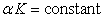(1)

Differentiating Eq. (1) with respect to volume V at constant pressure P: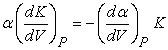(2)

which yields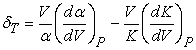using Eq.(2) or(3)

Where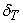is known as Anderson Gruneisen parameter at temperature T which is basically a measurement of anharmonicity in a crystal.

A linear relationship between bulk modulus (K) and temperature (T) has been reported by Fang  in the following way: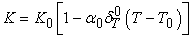(4)

where K,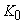,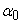andare isothermal bulk modulus at temperature T, bulk modulus at(i.e. at room temperature (reference temperature)), volume thermal expansion coefficient at room temperature and Anderson Gruneisen parameter at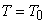.

Differentiating Eq.(4) with respect to T at constant P: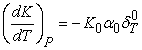(5)

From Eqs.(3) and (5), we get:(6)

Anderson Gruneisen parameter has been expressed as[32, 33] :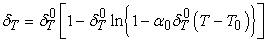(7)

Using Eqs. (4) and (7), Eq.(6) takes the form as:(8)

Integrating Eq. (8), we get: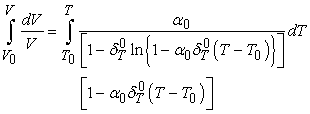(9)

On rearranging the terms, which yields:(10)

where.

The above Eq. (10) is newly established Integral Form of Equation of State (IFEOS) for volume thermal expansion for solids or nanocrystals. Since this new form of equation of state (EOS) has been developed by the authors of this paper Mahipal Singh & Madan Singh, therefore this EOS would be known as Singh & Singh Equation of State for volume thermal expansion. The beauty of this equation of state is that this equation requires only two input parametersand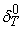at room temperature (reference temperature) and the value ofis evaluated directly as a function of temperature while the other integral form of equations of state involve more input parameters . This equation is also easier to handle in comparison of already existing integral form of equation of state (IFEOS). This newly developed EOS is independent of the crystal structure of the material under investigation. Therefore, it can expectedly be applied to study temperature dependent volume expansion of a wide variety of materials.

The following expression for temperature dependence of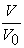has been obtained by Fang as: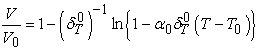(11)

The values of volume thermal expansion as a function of temperature for different nanomaterials fullerene (C60), 20 nm-Ni, 15 nm-(80Ni+20Fe), n-ZnO, n-TiO2 and n-NiO have been calculated by Eq. (10). The theoretical results obtained by Singh & Singh EOS have been compared with the results obtained by Eq. (11) and other existing experimental results.

### 3. Results and Discussion

The values of volume thermal expansion (), at different temperatures, have been calculated using newly established Singh and Singh equation of state for volume thermal expansion Eq. (10) and Eq. (11) as obtained by Fang  for fullerene (C60), 20 nm-Ni, 15 nm-(80Ni+20Fe), n-ZnO, n-TiO2 and n-NiO nanomaterials. These equations need only two input parameters- Anderson Gruneisen parameter () and volume thermal expansion coefficient () at room temperature (reference temperature). The input parameters used in this theoretical work are given in Table 1.

#### Table 1. Input parameters used in the present workDownload asPowerPoint Slide

Veiw figureView current table in a new window

The calculated values of volume thermal expansion () with temperature (T) for fullerene (C60), 20 nm-Ni, 15 nm-(80Ni+20Fe), n-ZnO, n-TiO2 and n-NiO nanomaterials using Eq. (10) are plotted in Figure 1 – Figure 6 and compared with theoretical  (as obtained by Eq. (11)) and experimental data [17, 23] for the sake of comparison of our theoretical results. These all figures reveal increasing trends with temperature increment. It is obvious that our calculated results of volume thermal expansion () for various nanomaterials are in excellent agreement to the existing experimental [17, 23] and theoretical results . Thus it is emphasized here that our integral form of equation of state (Eq. 10) successfully explains the volume thermal expansion of nanomaterials which supports the validity of Singh & Singh integral form of equation of state (IFEOS).

### 4. Conclusion

In the present work, a new integral form of equation of state (IFEOS) for volume thermal expansion has been established which is named as Singh & Singh equation of state. The values of volume thermal expansion () at different temperatures for a wide variety of nanomaterials C60, 20 nm-Ni, 15 nm-(80Ni+20Fe), n-ZnO, n-TiO2 and n-NiO have been calculated using this newly established Singh & Singh EOS. Our calculated results of volume thermal expansion () are found to be in excellent agreement to the existing experimental [17, 23] and theoretical results . It may be emphasized that our EOS (Singh & Singh EOS) explains the volume thermal expansion of nanomaterials successfully which supports the validity of Singh & Singh IFEOS.

### References

  A. S. Edelstein, R. C. Cammarata, Nanomaterials: Synthesis, Properties and Applications (Institute of Physics, Bristol, England), 1996.In article  H. W. Kroto, J. R. Heath, S. C. O’Brien, R. F. Curl, R. E. Smalley, Nature, 318 (1985) 162.In article CrossRef  J. E. Fischer, P. A. Heiney, A. R. Mcghie, W. J. Romanow, A. M. Denenstein, J. P. Mccauley, A.B. Smith III, Science, 252 (1991) 1288.In article CrossRef PubMed  A. Lundin, B. Sundquist, P. Skoglund, A. Fransson, S. Pettersson, Solid State Commun., 84 (1992) 879.In article CrossRef  E. Bonetti, E. G. Campari, L. Pasquini, J. Appl. Phys., 84 (1998) 4219.In article CrossRef  B. Chain, D. Penwell, M. B. Kruger, Solid State Commun., 115 (2000) 191.In article CrossRef  B, Sundqvist, Solid State Commun., 37 (1981) 289.In article  K. W. Katahara, M. H. Manghnani, E. S. Fisher, J. Phys. F: Met. Phys., 9 (1979) 773.In article CrossRef  K. Kusaba, Y. Syono, T. Kikegawa, Proc. Jpn. Acad. B, 75 (1999) 1.In article CrossRef  V. L. Solozhenko, O. O. Kurakevich, P. S. Sokolov, A. N. Baranov, J. Phys. Chem. A, 115 (2011) 4354.In article CrossRef PubMed  D. C. Look, Mater. Sci. Eng. B, 80 (2001) 383.In article CrossRef  J. S. Lee, K. Park, Journal of Crystal Growth, 254 (3-4) (2003) 423.In article CrossRef  P. H. Borse, L. S. Kankate, F. Dassenoy, W. Vogel, Journal of Materials Science, 13 (2002) 553.In article  V. Biju, A. M. Khadar, Mater. Sci. Eng. A, 304 (2001) 814.In article CrossRef  S. C. Kim, T. H. Kwon, J. Phys. Chem. Soilds, 53 (1992) 539.In article CrossRef  Y. Kuru, M. Wohlschlogel, U. Welzel, E. J. Mittemeijer, Applied Physics Letters, 90 (2007) 243113.In article CrossRef  R. Seelabouina, N. Phatak, R. P Gulve, H. P. Leirmann, S. K. Saxena, Thermal Conduct., 27 (2005) 647.In article  Jeewan Chandra, Deepika Kandpal, B. R. K. Gupta, High Temp-High Press, 37 (2008) 325.In article  Jeewan Chandra, Deepika Kandpal, B. R. K. Gupta, Physica B, 404 (2009) 1087.In article CrossRef  A. K. Srivastava, B. R. K. Gupta, Indian J of Pure and Appl. Phys., 46 (2008) 406.In article  K. Y. Singh, B. R. K. Gupta, Physica B, 334 (2003) 266.In article CrossRef  Uma D Sharma, H. Joshi, M. Kumar, Indian J of Pure & Appl. Phys., 48 (2010) 663.In article  Y. K. Kwon, S. Berber, D. Tomanek, Phys. Rev. Lett., 92 (2004) 015901.In article CrossRef PubMed  M. Born, K. Huang, Dynamical Theory of Crystal Lattice (Oxford University Press, Oxford) 1954.In article PubMed  S. S. Bedi, M. Singh, J. Singh, Solid State Commun. 89 (1994), 265.In article CrossRef  M. Ross, Phys. Rev. B, 21 (1980) 3140.In article CrossRef  P. Kuchchal, N. Dass, Pramana J. Phys., 52 (1999) 75.In article  G. Parsafar, J. A. Mason, Phys. Rev. B, 19 (1994) 3049.In article CrossRef  P. Vinet, J. R. Smith, J. Ferrante, J. H. Rose, Phys. Rev. B, 35 (1987) 1945.In article CrossRef  O. L. Anderson, K Zou, Phys. Chem. Miner, 16 (1989) 642.In article  Z. H. Fang, Physica B, 357 (2005) 433.In article CrossRef  N. Chuanhui, S. Huang, W. Huang, Applied Physics Research, 2 (1) (2010) 144.In article  Mahipal Singh, International J. of Phys. & Research, 3 (4) (2013) 55.In article  T Turi, U. Erb, Mater. Sci. Eng. A, 204 (1995) 34.In article CrossRef